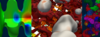The master's programme Computational Science and Engineering is suitable for graduates of a scientific or technical bachelor's programme.

Such bachelor's programmes at TU Wien are Civil Engineering, Electrical Engineering and Information Technology, Media Informatics and Visual Computing, Medical informatics, Software & Information Engineering, Computer Engineering, Mechanical Engineering, Technical Chemistry, Statistics and Mathematical Methods in Economics, Financial and Actuarial Mathematics, Technical Physics, Process Engineering, Geodesy and Geoinformation, Business Informatics and Mechanical Engineering - Management.

Other programmes are suitable as well, as long as they compare to one of the listed bachelor's programmes. In particular a programme is suitable if the following knowledge, skills and competencies amount to at least 30 ECTS:

## Computer Science

• Programming languages (at least one): C; C++; Fortran; Java; Python.
• Topics: Data Structures; Algorithms; Functions; Classes; Objectoriented Programming; Computer Architectures.

## Mathematics

• Linear algebra with eigenvalue problems: matrix and vector calculus; inverting matrices; solving linear systems of equations; determination of determinants; eigenvalues and eigenvectors
• Differential and integral calculus in one and several variables: real and complex numbers; concept of function; sequences and series; derivation in a variable; integration in a variable; power series; gradients and total derivative; simple multidimensional domain integrals (rectangles, triangles)
• Ordinary differential equations: linear differential equations; solution by means of separation of the variables